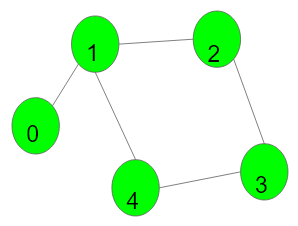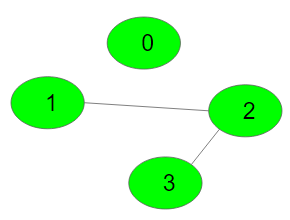XDAYS

:

HOUR

:

MINS

:

SEC

Copied to Clipboard
Detect cycle in an undirected graph
Medium Accuracy: 35.66% Submissions: 100k+ Points: 4

Given an undirected graph with V vertices and E edges, check whether it contains any cycle or not.Example 1:

Input:
V = 5, E = 5
adj = {{1}, {0, 2, 4}, {1, 3}, {2, 4}, {1, 3}}
Output: 1
Explanation:1->2->3->4->1 is a cycle.


Example 2:

Input:
V = 4, E = 2
adj = {{}, {2}, {1, 3}, {2}}
Output: 0
Explanation:No cycle in the graph.


You don't need to read or print anything. Your task is to complete the function isCycle() which takes V denoting the number of vertices and adjacency list as input parameters and returns a boolean value denoting if the undirected graph contains any cycle or not, return 1 if a cycle is present else return 0.

NOTE: The adjacency list denotes the edges of the graph where edges[i] stores all other vertices to which ith vertex is connected.

Expected Time Complexity: O(V + E)
Expected Space Complexity: O(V)

Constraints:
1 ≤ V, E ≤ 105

### Editorial

We strongly recommend solving this problem on your own before viewing its editorial. Do you still want to view the editorial?

#### My Submissions: